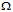# Digital Electronics - Digital Signal Processing

### Exercise :: Digital Signal Processing - Filling the Blanks

6.

The principal advantage of the three-wire handshake on the GPIB is that it ________.

 A. is faster than two-wire methods B. allows fast and slow listeners on the same bus C. can be used over very long distances D. is compatible with the RS232C standard

Explanation:

No answer description available for this question. Let us discuss.

7.

An ADC that uses an up/down counter (and other devices) to follow changes in the input analog signal is a ________.

 A. single-slope ramp converter B. dual-slope ramp converter C. successive-approximation converter D. tracking converter

Explanation:

No answer description available for this question. Let us discuss.

8.

The number of data lines on the GPIB is ________.

 A. 1 B. 4 C. 8 D. 16

Explanation:

No answer description available for this question. Let us discuss.

9.

The resolution of a DAC can be expressed as the ________.

 A. minimum output voltage B. number of bits that are converted to an analog output C. deviation of the output from a straight line D. difference between the expected and actual outputs

Explanation:

No answer description available for this question. Let us discuss.

10.

Assume that in a certain 4-bit weighted ladder DAC, the input representing the most significant bit is applied to a 20 kresistor. What is the size of the resistor that represents the least significant bit?

 A. 2.5 kB. 5 kC. 80 kD. 160 k